# CBSE Class 12th Chemistry Question Paper Solved Last 10 Years With Solution

Here we are providing CBSE Previous Year Question Papers Class 6 to 12 solved with soutions CBSE Class 12th Chemistry Question Paper Solved Last 10 Years with Solution Chemistry sample paper class 12, Chemistry previous year question paper class 12, cbse class 12 Chemistry sample paper, cbse class 12 Chemistry sample paper 2020, Chemistry sample paper class 12 2020, cbse sample paper 2020 class 12 Chemistry, class Practice of previous year question papers and sample papers protects each and every student to score bad marks in exams.If any student of CBSE Board continuously practices last year question paper student will easily score high marks in tests. Fortunately earlier year question papers can assist the understudies with scoring great in the tests. Unraveling previous year question paper class 12 Chemistry is significant for understudies who will show up for Class 12 Board tests.

## Class 12 Subject Chemistry Paper Set 5 with Solutions

SECTION : A

Read the given passage and answer the questions 1 to 5 that follow : The halogens have the smallest atomic radii in their respective periods. The atomic radius of fluorine is extremely small. All halogens exhibit – 1 oxidation state. They are strong oxidising agents and have maximum negative electron gain enthalpy. Among halogens, fluorine shows anomalous behaviour in many properties. For example electro negativity and ionisation enthalpy are higher for fluorine than expected whereas bond dissociation enthalpy, m.p. and b.p. and electron gain enthalpy are quite lower than expected. Halogens react with hydrogen to give hydrogen halides (HX) and combine amongst themselves to form a number of compounds of the type XX’, XX’3, XX’5 and XX’7 called inter-halogens.

Question 1: (Marks 1)

Why halogens have maximum negative electron gain enthalpy ?

Answer :

By gaining one electron they acquire noble gas configuration/ smallest size and high effective nuclear charge in their respective period.

Question 2: (Marks 1)

Why fluorine shows anomalous behaviour as compared to other halogens ?

Answer :

Extremely small size/ absence of d orbital/highest electronegativity / low bond dissociation enthalpy of F-F bond.

Question 3: (Marks 1)

Arrange the hydrogen halides (HF to HI) in the decreasing order of their reducing character.

Answer :

HI>HBr>HCl>HF

Question 4: (Marks 1)

Why fluorine is a stronger oxidizing agent than chlorine ?

Answer :

Low bond dissociation enthalpy and high hydration enthalpy.

Question 5: (Marks 1)

What are the sizes of X and X’ in the interhalogen compounds ?

Answer :

X >X’ /X is bigger in size and X’ is smaller.

Question 6 to 10 are one word answers.

Question 6: (Marks 1)

Name the cell used in hearing aids and watches.

Answer :

Mercury cell

Question 7: (Marks 1)

How much charge in terms of Faraday is required to reduce one mol of MnO4 to Mn2+?

Answer :

5 F

Question 8: (Marks 1)

Write the slope value obtained in the plot of log [Ro] / [R] Vs. time for a first order reaction.

Answer :

k/2.303

Question 9: (Marks 1)

Name the sweetening agent used in the cooking of sweets for a diabetic patient.

Answer :

Saccharine/Sucralose / alitame (any other except Aspartame)

Question 10: (Marks 1)

Name the polymer which is used for making electrical switches and combs.

Answer :

Bakelite

Questions 11 to 15 are multiple choice questions.

Question 11: (Marks 1)

In the Mond’s process the gas used for the refining of a metal is

(a) H2

(b) CO2

(c) CO

(d) N2

Answer :

(c) CO

Question 12: (Marks 1)

The conversion of an alkyl halide into an alcohol by aqueous NaOH is classified as

(a) a dehydrohalogenation reaction

(b) a substitution reaction

(c) an addition reaction

(d) a dehydration reaction

Answer :

(b) a substitution reaction

Question 13: (Marks 1)

CH3CONH2 on reaction with NaOH and Br2 in alcoholic medium gives

(a) CH3CH2NH2

(b) CH3CH2Br

(c) CH3NH2

(d) CH3COONa

Answer :

(c) CH3NH2

Question 14: (Marks 1)

The oxidation state of Ni in [Ni(CO)4 ] is

(a) 0

(b) 2

(c) 3

(d) 4

Answer :

(a) 0

Question 15: (Marks 1)

Amino acids are

(a) acidic

(b) basic

(c) amphoteric

(d) neutral

Answer :

One mark may be awarded to any option

Questions 16 to 20.

(A) Both Assertion (A) and Reason (R) are correct statements, and Reason (R) is the correct explanation of the Assertion (A).

(B) Both Assertion (A) and Reason (R) are correct statements, but Reason (R) is not the correct explanation of the Assertion (A).

(C) Assertion (A) is correct, but Reason (R) is wrong statement.

(D) Assertion (A) is wrong, but Reason (R) is correct statement.

Question 16: (Marks 1)

Assertion (A) : Conductivity of an electrolyte increases with decrease in concentration.

Reason (R) : Number of ions per unit volume decreases on dilution.

Answer :

(D) Assertion (A) is wrong, but Reason (R) is correct statement.

Question 17: (Marks 1)

Assertion (A) : The C-O-C bond angle in ethers is slightly less than tetrahedral angle.

Reason (R) : Due to the repulsive interaction between the two alkyl groups in ethers.

Answer :

(D) Assertion (A) is wrong, but Reason (R) is correct statement.

Question 18: (Marks 1)

Assertion (A) : Low spin tetrahedral complexes are rarely observed.

Reason (R) : Crystal field splitting energy is less than pairing energy for tetrahedral complexes.

Answer :

(A) Both Assertion (A) and Reason (R) are correct statements, and Reason (R) is the correct explanation of the Assertion (A).

Question 19: (Marks 1)

Assertion (A) : Elevation in boiling point is a colligative property.

Reason (R) : Elevation in boiling point is directly proportional to molarity.

Answer :

(C) Assertion (A) is correct, but Reason (R) is wrong statement.

Question 20: (Marks 1)

Assertion (A) : Oxidation of ketones is easier than aldehydes.

Reason (R) : C-C bond of ketones is stronger than C-H bond of aldehydes.

Answer :

(D) Assertion (A) is wrong, but Reason (R) is correct statement.

SECTION – B

Question 21: (Marks 2)

State Raoult’s law for a solution containing volatile components. What is the similarity between Raoult’s law and Henry’s law ?

Answer :

► For a solution of volatile liquids, the partial vapour pressure of each component of the solution is directly proportional to its mole fraction present in solution.

► If we compare the equations for Raoult’s law and Henry’s law, it can be seen that the partial pressure of the volatile component or gas is directly proportional to its mole fraction in solution.

Question 22: (Marks 2)

Write the role of

(a) Dilute NaCN in the extraction of Gold.

(b) CO in the extraction of Iron.

OR

How is leaching carried out in the case of low grade copper ores ? Name the method used for refining of copper metal.

Answer :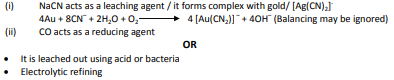Question 23: (Marks 2)

Define adsorption with an example. What is the role of adsorption in heterogeneous catalysis ?

OR

Define Brownian movement. What is the cause of Brownian movement in colloidal particles ? How is it responsible for the stability of Colloidal Sol ?

Answer :

► The accumulation of molecular species at the surface rather than in the bulk of a solid or liquid. Example: adsorption of gases on surface of active charcoal (or any other suitable example)

► Adsorption of reactants occurs on surface of catalyst and reaction takes place.

OR

► A state of continuous zig-zag motion of particles.

► Unbalanced bombardment of the particles by the molecules of the dispersion medium.

► The Brownian movement has a stirring effect which does not permit the particles to settle.

Question 24: (Marks 2)

(a) Write the IUPAC name and hybridisation of the complex [Fe(CN)6]3–.

(Given : Atomic number of Fe = 26)

(b) What is the difference between an ambidentate ligand and a chelating ligand ?

Answer :

(a) Hexacyanidoferrate(III) / Hexacyanoferrate(III)

d2sp3

(b) Ligand which can ligate through two different atoms is called ambidentate ligand whereas di- or polydentate ligand uses its two or more donor atoms to bind a single metal ion. / a chelating ligand forms a more stable complex as compared to an ambidentate ligand. / chelating ligand forms a cyclic complex while ambidentate ligand forms a non-cyclic complex.

Question 25: (Marks 2)

How do antiseptics differ from disinfectants ? Name a substance which can be used as a disinfectant as well as an antiseptic.

Answer :

► Antiseptic is applied on living tissue, to kill or stop growth of microbes while disinfectant is applied on inanimate/ non -living objects

► 0.2 per cent solution of phenol is an antiseptic while its one percent solution is disinfectant.

Question 26: (Marks 2)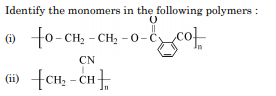Answer :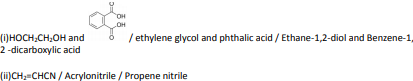Question 27: (Marks 2)

Draw the structures of the following :

(i) H2S2O8

(ii) XeF6

Answer :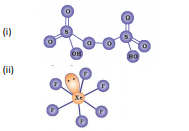SECTION : C

Question 28: (Marks 3)

A 0.01 m aqueous solution of AlCl3 freezes at – 0.068 °C. Calculate the percentage of dissociation. [Given : Kf for Water = 1.86 K kg mol–1].

Answer :

ΔTf = iKf m
0.068 = i x 1.86 x 0.01
i = 3.65 or 3.656
α = i-1/n-1
α = 0.883 or 0.885
88.3% or 88.5%

Question 29: (Marks 3)

When a steady current of 2A was passed through two electrolytic cells A and B containing electrolytes ZnSO4 and CuSO4 connected in series, 2 g of Cu were deposited at the cathode of cell B. How long did the current flow ? What mass of Zn was deposited at cathode of cell A ?

[Atomic mass : Cu = 63.5 g mol–1, Zn = 65 g mol–1; 1F = 96500 C mol–1]

Answer :

m=Z I t
2 = 63.5 x 2 x t/2x96500
t = 3039.4 s
m1/m2 = eq wt 1/eq wt 2
2 / m2 = 63.5/2 / 65/2
m2 = 2.05 g

Question 30: (Marks 3)

Differentiate between following :

(i) Amylose and Amylopectin

(ii) Globular protein and Fibrous protein

(iii) Nucleotide and Nucleoside

Answer :

(i) Amylose is water soluble component of starch while amylopectin is insoluble in water

(ii) Globular proteins are spherical in shape while fibrous are linear.

(iii) Nucleoside consists of a sugar and a base

When nucleoside is linked to phosphate group, it forms a nucleotide

Question 31: (Marks 3)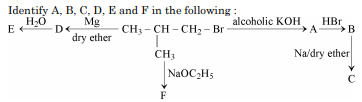Answer :

A: ( CH3 )2 C=CH2

B : ( CH3 )2 CBrCH3

C : ( CH3 )3 C - C(CH3 )3

D: ( CH3 )2 CHCH2MgBr

E : ( CH3 )2 CHCH3

F: ( CH3 )2 CHCH2OC2H5

Question 32: (Marks 3)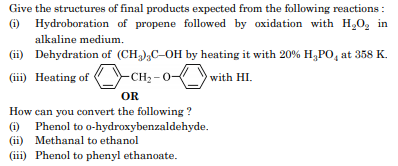Answer :Question 33: (Marks 3)

Give reasons :

(i) Aniline does not undergo Friedal-Crafts reaction.

(ii) Aromatic primary amines cannot be prepared by Gabriel’s phthalimide synthesis.

(iii) Aliphatic amines are stronger bases than ammonia.

Answer :

(i) Aniline forms salt with AlCl3, the Lewis acid.

(ii) Aryl halides do not undergo nucleophilic substitution with the anion formed by phthalimide

(iii) Due to +I effect of alkyl group electron density on N atom increases.

Question 34: (Marks 3)

Write three differences between lyophobic sol and lyophilic sol.

OR

Define the following terms :

(i) Protective colloid

(ii) Zeta potential

(iii) Emulsifying agent

Answer :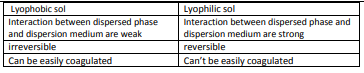OR

(i) Lyophilic colloids have a unique property of protecting lyophobic colloids./ Lyophilic colloids form a layer around the lyophobic colloids to protect them from the electrolyte or coagulation.

(ii) Potential difference between the fixed layer and the diffused layer of opposite charges of a colloid.

(iii) Substances used for stabilisation of an emulsion.

SECTION : D

Question 35: (Marks 5)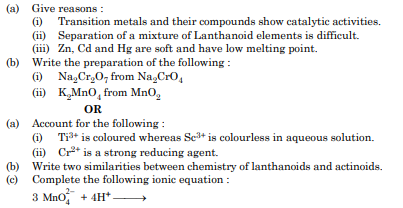Answer :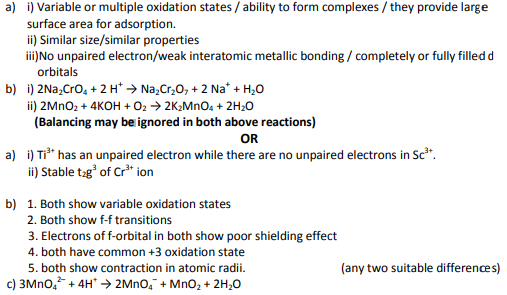Question 36: (Marks 5)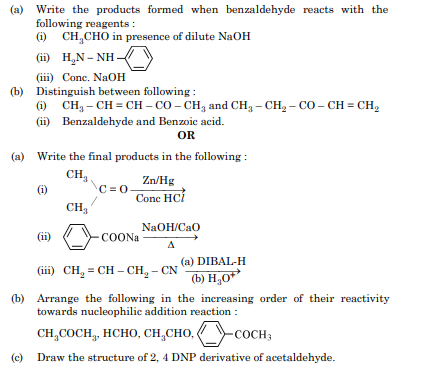Answer :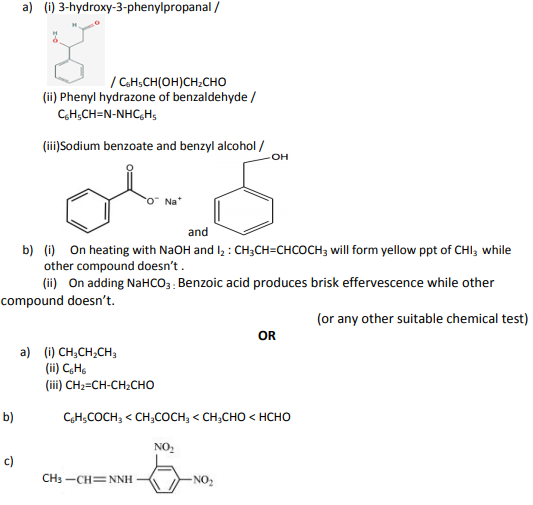Question 37: (Marks 5)

(a) A first order reaction is 25% complete in 40 minutes. Calculate the value of rate constant. In what time will the reaction be 80% completed ?

(b) Define order of reaction. Write the condition under which a bimolecular reaction follows first order kinetics.

OR

(a) A first order reaction is 50% complete in 30 minutes at 300 K and in 10 minutes at 320 K. Calculate activation energy (Ea) for the reaction. (R = 8.314 J K–1 mol–1)

(b) Write the two conditions for collisions to be effective collisions.

(c) How order of reaction and molecularity differ towards a complex reaction ?

[Given : log 2 = 0.3010, log 3 = 0.4771, log 4 = 0.6021, log 5 = 0.6991]

Answer :

a) k = (2.303 / t) log ([A]o / [A]t)

k = (2.303 / 40) log (100 / 75)

= 0.007 min-1 or 0.0071 min-1 or 0.0072 min-1

t = (2.303 / k) log ([A]o / [A]t)

t = (2.303 / 0.0071) log (100/20)

t =230 min or 226.7min or 223.7 min.

b) Sum of powers of the concentration of the reactants in the rate law expression. When one of the reactant is present in large excess.

OR

a) k = 0.693/ t1/2

k1= 0.693/ t1/2= 0.693 / 30

k2= 0.693/ t1/2= 0.693 / 10

log k2/k1 = Ea /2.303 R (1/ T1 -1/ T2)

log 3 = Ea /2.303 x 8.314 (1/ 300 -1/ 320)

Ea = 2.303 X 8.314 x 0.4771 x ( 300 x 320/20)

= 43848.5 J/mol OR 43855 J/mol or 43.8 kJ/mol

b) Proper orientation Energy of the colliding particles should be more than threshold energy

c) For a complex reaction, order of reaction is applicable while molecularity has no meaning.

☞ Click here for privious year Question papers

## Chemistry sample paper class 12, Chemistry previous year question paper class 12, cbse class 12 Chemistry sample paper, cbse class 12 Chemistry sample paper 2020, Chemistry sample paper class 12 2020, cbse sample paper 2020 class 12 Chemistry, class 12 Chemistry sample paper 2020, class 12 important questions Chemistry, cbse class 12 board exam Chemistry paper, Chemistry previous year question papers class 12 with solutions, Chemistry sample paper class 12 2019, cbse class 12 Chemistry question paper 2017 solved pdf, cbse class 12 Chemistry question paper 2018, class 12 Chemistry paper 2019, Chemistry question paper for class 12, cbse class 12 Chemistry paper 2019

### NCERT Books Free Pdf Download for Class 5, 6, 7, 8, 9, 10 , 11, 12 Hindi and English Medium

 Mathematics Biology Psychology Chemistry English Economics Sociology Hindi Business Studies Geography Science Political Science Statistics Physics Accountancy

## Please Share this webpage on facebook, whatsapp, linkdin and twitter.

Copyright @ ncerthelp.com A free educational website for CBSE, ICSE and UP board.